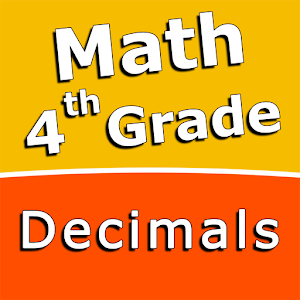\$2.99

# Android Giveaway of the Day - Fourth grade Math skills - Decimals

A choice of three fun and engaging mini games in addition to a regular math trainer mode!
\$2.99 期限切れ
ユーザーの評価: 0 (0%) 0 (0%)

このgiveawayオファーはすでに期限が切れています。Fourth grade Math skills - Decimalsが今紹介されています。

Intuitive interface powered by handwriting input and a choice of three fun and engaging mini games in addition to a regular math trainer mode make our app stand out from the crowd of generic math learning apps.

With Fourth grade Math skills - Decimals you can practice and improve the following math skills:
- Subtract decimal numbers
- Convert decimals to fractions and mixed numbers
- Convert fractions and mixed numbers to decimals - denominators of 10 and 100
- Convert fractions and mixed numbers to decimals
- Round decimals to the nearest whole number
- Round decimals to the nearest tenth
- Round decimals to the nearest hundredth

Choose between four different modes:
- Math Trainer
- Math Shot mini-game
- Math Gravity mini-game
- Math Trick Shot mini-game

Sergey Malugin

Education

1.0.0

11M

Everyone

4.0 and up

## コメント Fourth grade Math skills - Decimals

Thank you for voting!